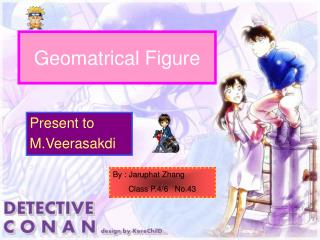DownloadDownload PresentationGeomatrical Figure

# Geomatrical Figure

Download Presentation## Geomatrical Figure

- - - - - - - - - - - - - - - - - - - - - - - - - - - E N D - - - - - - - - - - - - - - - - - - - - - - - - - - -
##### Presentation Transcript

1. Geomatrical Figure Present to M.Veerasakdi By : Jaruphat Zhang Class P.4/6 No.43

3. Rectangle A rectangle is a four-side shape where every angle is a right angle(90°). Also opposite sides are parallel and of equal length.

4. Square A square has equal sides and every angle is right angle (90º) Also opposite sides are parallel.

5. Parallelogram Opposite sides are parallel and equal in length, and opposite angles are equal (angle “a” are the same, and angles “b” are the same)

6. Rhombus A rhombus is a four-sided shape where all sides have equal length. Also opposite side are parallel amd opposite angles are equal.

7. Triangles There are several different types of triangles. You can classify a triangle by its sides and its angles. There are THREE different classifications for triangles based on their sides. There are FOUR different classifications for triangles based on their angles.

8. Classifying Triangles by Sides EQUILATERAL - 3 congruent sides ISOSCELES – at least two sides congruent SCALENE – no sides congruent

9. Classifying Triangles by Angles • EQUIANGULAR – all angles are congruent • ACUTE – all angles are acute • RIGHT – one right angle • OBTUSE – one obtuse angle

10. Can You Classify the Different Triangles in the Picture Below? Classify the following triangles : AED, ABC, ACD, ACE

11. The Classifications… • Triangle AED = Equilateral, Equiangular • Triangle ABC = Equilateral, Equiangular • Triangle ACD = Isoceles, Obtuse • Triangle ACE = Scalene, Right • So how did you do?